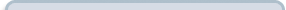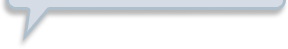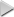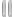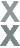# Fan Appreciation Night, Part 1

###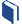Resources for this lesson: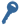Key Term

Complement

> Glossary> Calculator Resources> Teacher Resources: Instructional Notes## Test and Confirm

Let’s practice with a few more examples.  Write solutions as a decimal rounded to the nearest thousandth.

##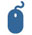Check Your Understanding

When you finish this activity, scroll down the page.

Probabilities of the complement of an event may also be found. In probability, the complement of an event is a list of all outcomes that are not the desired outcome for an event. There are several notations used for the probability of the complement of an event. The notations that you may see for the probability of the complement of A are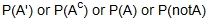. In this lesson the symbol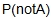is used when discussing the probability of an event not occurring.

To find the probability of an event not occurring, calculate the probability of an event occurring and then subtract the event from 1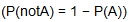. So if event A is drawing a history card on the first draw, then the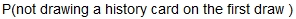, 1 $-\frac{1}{6}=\frac{5}{6}$.

##Check Your Understanding

When you finish this activity, scroll down the page.